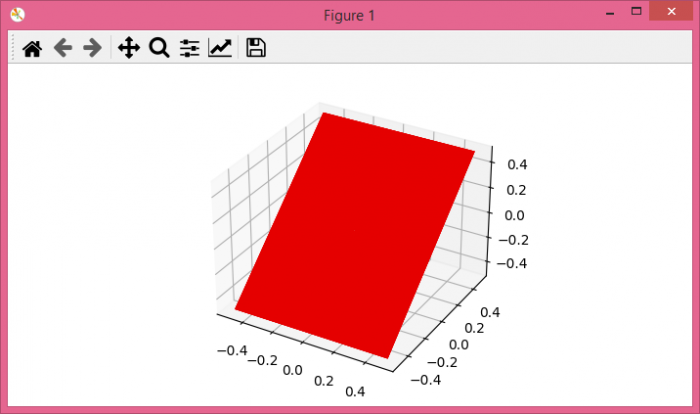# Plotting a masked surface plot using Python, Numpy and Matplotlib

To plot a masked surface plot using Python, Numpy and Matplotlib, we can take the following steps −

• Set the figure size and adjust the padding between and around the subplots.
• Create a new figure or activate an existing figure.
• Add an 'ax' to the figure as part of a subplot arrangement.
• Return the coordinate matrices from coordinate vectors, pi and theta.
• Create x, y and z with masked data points.
• Create a surface plot with x, y, and z data points.
• To display the figure, use show() method.

## Example

import matplotlib.pyplot as plt
import numpy as np

plt.rcParams["figure.figsize"] = [7.00, 3.50]
plt.rcParams["figure.autolayout"] = True

fig = plt.figure()
pi, theta = np.meshgrid(
np.arange(1, 10, 2) * np.pi / 4,
np.arange(1, 10, 2) * np.pi / 4)

x = np.cos(pi) * np.sin(theta)
y = np.sin(pi) * np.sin(theta)
z = np.ma.masked_where(x >= 0.01, y)

ax.plot_surface(x, y, z, color='red')

plt.show()

## Output

It will produce the following output